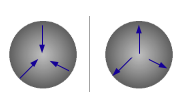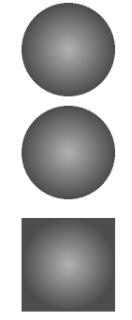## Thursday, 26 May 2011

### Understanding the Image Reflection - Gradient

This is my second post about image reflection. In our first post we discussed about basic principles of image inversion. We discussed translation, scaling and bitmap context to understand image inversion. Today we will discuss about gray scale images and gradients. These concepts are important not only to understand reflection but these could also be used in other scenarios. Our main goal is to understand image reflection which is implemented in Reflection sample provided by Apple. All examples which we are going to discussed today can be downloaded from github repository. If you are looking for complete example of Reflection which covers image inversion, gradient and masking then I would refer you to my post about image masking.

Gray Scale images:
As we know pixels are basic elements of digital image. Each pixel contains number of values which determine the type of image. There are mainly three types of images: black & white, gray scale and color. Black & white images are also called binary images as each pixel has a value either 0 or 1. The next complex type is a gray scale image where each pixel stores value of gray level or gray scale. For example if an image is 8-bit grayscale then each pixel can contain value between 0 to 256 inclusive.
The gray scale images are black & white images but with shades of gray. We can also think shades of gray as color intensities where 0 means black and 1 means white. There are several uses of gray scale images in digital image processing specially in medical image analysis. One common example which we have all come across is X-rays. Here is an example of how we can create a gray scale image from a color image:
```UIImage* createGrayScaleImage(UIImage* originalImage){
//create gray device colorspace.
CGColorSpaceRef space = CGColorSpaceCreateDeviceGray();
//create 8-bit bimap context without alpha channel.
CGContextRef bitmapContext = CGBitmapContextCreate(NULL, originalImage.size.width, originalImage.size.height, 8, 0, space, kCGImageAlphaNone);
CGColorSpaceRelease(space);
//Draw image.
CGRect bounds = CGRectMake(0.0, 0.0, originalImage.size.width, originalImage.size.height);
CGContextDrawImage(bitmapContext, bounds, originalImage.CGImage);
//Get image from bimap context.
CGImageRef grayScaleImage = CGBitmapContextCreateImage(bitmapContext);
CGContextRelease(bitmapContext);
//image is inverted. UIImage inverts orientation while converting CGImage to UIImage.
UIImage* image = [UIImage imageWithCGImage:grayScaleImage];
CGImageRelease(grayScaleImage);
return image;
}
```
In this function first we receive color image as a parameter. We convert this image into gray scale image. However it is not necessary that we need to have an image before we can draw gray scale image. We can draw gray scale image using different draw functions without loading any image.  After loading image we create a gray scale color space. We use this color space to create a bitmap context of the size of original image. We are not using alpha channel in our context so we set it to kCGImageAlphaNone. Next we draw original image into gray scale bitmap context. We get the handle to this image by calling CGBitmapContextCreateImage. If you remember we discussed in our last post that the images drawn in bitmap context are in different orientation than UIView and CALayer. We use UIImage wrapper to adjust the orientation of newly created image. Here is the output of our function.Figure 1
As we mentioned earlier gray scale image represents intensity of color at each pixel. If we compare our new gray scale image with original color image we will notice that the body of bus which is red in original image and tyres which are green have different gray shades in gray scale image. Similarly smoke remains white as it has maximum intensity where as transparent background becomes black as it has no intensity in original image. So how does it help us to achieve image reflection? Basically in order to produce fade effect in image reflection, we will create a gray scale gradient and mask it with the original image to produce fade effect. We will discuss gradient in next section but we will discuss masking in our next post.

In Vector Algebra gradient is used to determine the direction and magnitude i.e vector field from scalar quantity. In terms of images, image gradient defines the variation and direction of color intensity at each point or region. We can illustrate it by simply drawing a gradient in image editing tool such as Gimp.Figure 2: (a) Rectangle (b) Rectangle after gradient

Figure 2 (a) shows rounded rectangle filled with black color. Figure 2(b) shows the effect of gradient on this rectangle. We can visually see that the intensity of color increases as it moves towards lower end of the rectangle and vice versa. Therefore in this case gradient is either from top to bottom or from bottom to top of the rectangle. There are different types of gradients. In iOS there are two types of gradient functions available. One is Linear gradient and the other is Radial gradient.

In a linear gradient color levels or intensities vary along an axis between two end points. The color levels or intensities perpendicular to that axis remains same. For example if we apply a gradient from top to bottom of a rectangle as shown in figure 2(b) then it varies along y-axis but it remains same along x-axis. In two dimensional coordinate system, linear gradient can be applied from top to bottom (y-axis), bottom to top(-y axis), left to right (x-axis) or right to left (- x axis). We will now see how it is implemented in code. The output of our code will be as follows:Figure 3: Linear Gradient (a) Rounded Rectangle (b) Rounded Rectangle after gradient.
Fig 3(a) shows rounded rectangle and fig 3(b) shows same rounded rectangle after being applied with gradient. If you notice after gradient fig 3(b) rounded rectangle gives a 3D look similar to iOS buttons and controls.
```//Draw gradient.
//start and end points of gradient.
CGPoint startPoint = CGPointMake(CGRectGetMidX(rect), CGRectGetMinY(rect));
CGPoint endPoint = CGPointMake(CGRectGetMidX(rect), CGRectGetMaxY(rect));
//locations of color defined in components.
CGFloat locations = {0.0, 1.0};
//color components array. In this case color is same i.e white but we are changing the alpha value.
CGFloat components = { 1.0, 1.0, 1.0, 0.0,
1.0, 1.0, 1.0, 0.7};
//RGB color space.
CGColorSpaceRef colorSpace = CGColorSpaceCreateDeviceRGB();
CGColorSpaceRelease(colorSpace);
}
```Figure 4 Radial Gradient (a) Direction towards centre point. (b) Direction away from centre point.
Now we will see how we can implement Radial gradient in iOS. Our example output would be as shown in figure 5.Figure 5: Radial gradient with different parameters. (a) Gradient with No Option (b) Gradient with kCGGradientDrawsBeforeStartLocation (c) Gradient with kCGGradientDrawsAfterEndLocation
This is how we generated our examples:
```- (void)drawRect:(CGRect)rect {
CGPoint startCenter, endCenter;
startCenter = endCenter = CGPointMake(CGRectGetMidX(rect), CGRectGetMidY(rect));
CGFloat endRadius = CGRectGetMidX(rect) > CGRectGetMidY(rect) ? CGRectGetMidX(rect): CGRectGetMidY(rect);
CGFloat locations = {0.0, 1.0};
//black color
CGFloat components = {0.0,0.0,0.0,0.3,
0.0, 0.0, 0.0, 0.7};
//Drawing code.
CGContextRef context = UIGraphicsGetCurrentContext();
//Get RGB color space
CGColorSpaceRef space = CGColorSpaceCreateDeviceRGB();
CGColorSpaceRelease(space);
}
```Figure 6: View with gradient.
1.